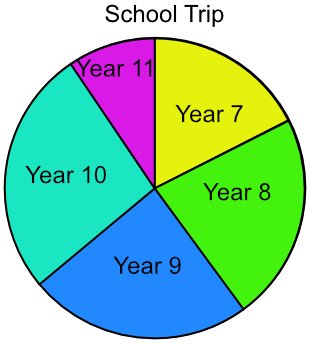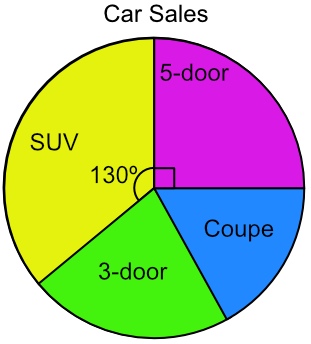Pie Charts

## Pie Charts

A Pie Chart shows data as sectors of a circle. A pie chart is built from a table of data.

Building a pie chart consists of these steps:

• Add the frequencies to obtain a total;

• Divide 360º by the total (to get the number of degrees for one item);

• Multiply each frequency by this number;

• Plot the pie chart using these values;

• Label the pie chart and provide a title

## Example 1

A school is taking 120 students on a trip. The students come from several year groups. Plot a pie chart to represent the data.

 Frequency Year 7 21 Year 8 27 Year 9 29 Year 10 32 Year 11 11

Add frequencies for total = 120.

Each student is worth 360 ÷ 120 = 3º

Multiply each frequency by 3º

 Frequency Year 7 21 3º x 21 = 63 Year 8 27 3º x 27 = 81 Year 9 29 3º x 29 = 87 Year 10 32 3º x 32 = 96 Year 11 11 3º x 11 = 33 Totals 120 360º

Plot the chart, adding a title and labels## Example 2

The pie chart below shows the type of cars sold by a car showroom over one month. 18 5-door cars were sold. How many SUVs were sold?18 five-door cars = 90º

1 car = 90 ÷ 18 = 5º for each car

SUVs = 130º ÷ 5 = 26 cars